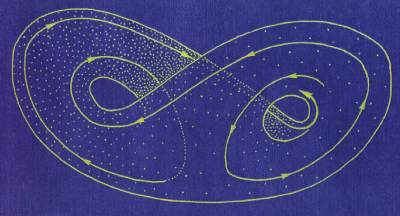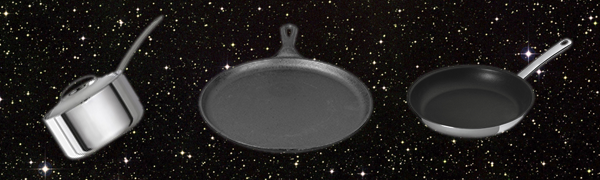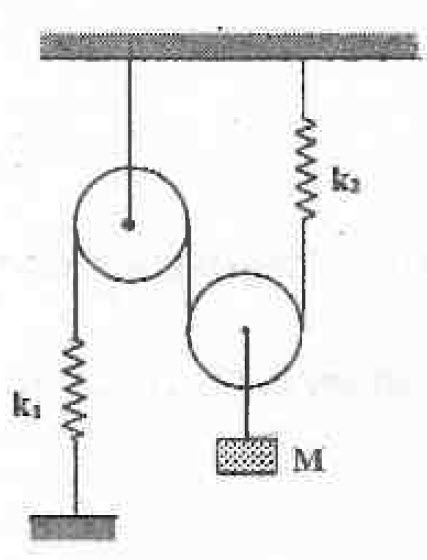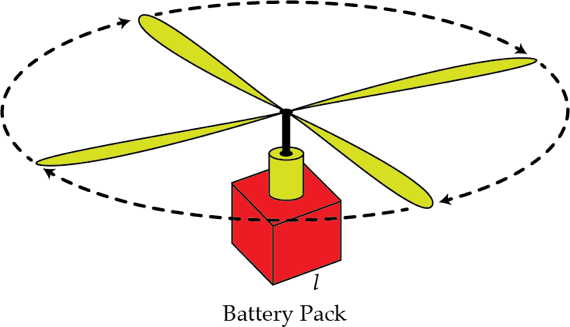Classical Mechanics

# Mechanics Warmups: Level 4 ChallengesA dynamical system is described by the equation $\dot{r} = f(r)$ where $f(r)$ is an $n^\text{th}$ order polynomial with roots $\{r_1,\ldots,r_n\}$. In other words, the roots of $f$ are the steady states of the system ($\dot{r}(r_i) = 0$).

Suppose the system is placed into one of the steady states $r_i$ and is perturbed very slightly away to $r_i+\Delta r$. How does the perturbation change over time?Three objects of same heat capacity with temperature $T_1=200~\mbox{K}$, $T_2=400~\mbox{K}$ and $T_3=400~\mbox{K}$ exchange heat with each other. They are isolated from the rest of the universe. Find the highest possible temperature one of them can reach in kelvin.

Details and assumptions:

• You may connect them to a heat engine and/or to a refrigerator
• The objects do not collide to generate heat energyFind the Time Period of oscillations (in $\displaystyle \text{sec}$) for the arrangement as shown in the figure.

Details and Assumptions:

• The pulleys are smooth and massless.
• $\displaystyle k_1 = 100 N/m$
• $\displaystyle k_2 = 200 N/m$
• $\displaystyle M = 4 kg$
• $\displaystyle g = 9.8 m/s^2$A dynamical system is described by the equation $\dot{r} = f(r)$ where $f(r)$ is an $n^\text{th}$ order polynomial with roots $\{r_1,\ldots,r_n\}$. In other words, the roots of $f$ are the steady states of the system ($\dot{r}(r_i) = 0$).

Suppose the system is placed into one of the steady states $r_i$ and is perturbed very slightly away to $r_i+\Delta r$. How does the perturbation change over time?

Toy helicopters with rechargeable batteries fly for a few minutes on a single charge. Manufacturers want to choose the right size of battery to achieve the longest flight time between charges. A larger battery stores more energy, but also increases the mass of the helicopter so it takes more energy to keep it in the air. Our question is, for a helicopter and battery type that behave as specified in the assumptions below, what is the linear size $l$ of the battery in mm that will maximize the time the helicopter can hover in place?Details and assumptions

• The acceleration of gravity is $-9.8~\mbox{m/s}^2$.
• The base mass $m_0$ of the helicopter without the battery is $50~\mbox{g}$.
• The battery is a uniform cube with side length $l$ and density $\rho= 4000 ~\mbox{kg/m}^3$.
• The total energy contained in the battery does not quite scale as the volume does, but instead scales as $l^{2.7}$.
• The emitted voltage from the battery is constant and does not change with $l$. All that changes is how long the battery lasts. Therefore the rotors spin and push air in the same way no matter what the battery size is.
• Assume the mass of the air pushed by the rotors is a constant value, independent of rotor speed. In an actual helicopter the mass increases with speed, effectively from Bernoulli's equation, but that's more complication than we want to go into.
×